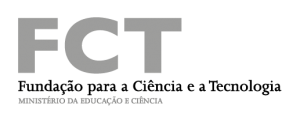# Quadratic and biquadratic extensions of \mathbb{F}_q((t)) and the local Langlands correspondence

Orador: Sérgio Mendes (ISCTE-IUL). Data, hora e local: 4 de Março de 2015, início às 14h30m na sala de reuniões do Departamento de Matemática.

Resumo: Let$\displaystyle K=\mathbb{F}_q((t))$ be a local function field of characteristic 2. Quadratic extensions in this case are Artin-Schreier extensions. The group$\displaystyle K/K^{2\times}$ is an infinite profinite group. As a consequence there are countable many quadratic and biquadratic extensions of$\displaystyle K$. We show that these extensions lead to a parametrization of certain$\displaystyle L$-packets – those with cardinality 2 and 4 – which play a fundamental role in the construction of the Langlands correspondence for the reductive group$\displaystyle SL_2(K)$. Every quadratic character comes from the Artin-Schreier symbol. Explicit formulae for quadratic characters are computed. If time permits we will relate these results with a recent geometric conjecture. The talk will be elementary and as self-contained as possible. Joint work with Roger Plymen.

Seminário realizado com o apoio do Centro de Matemática – 212 (Pest-OE/MAT/UI0212/2014).